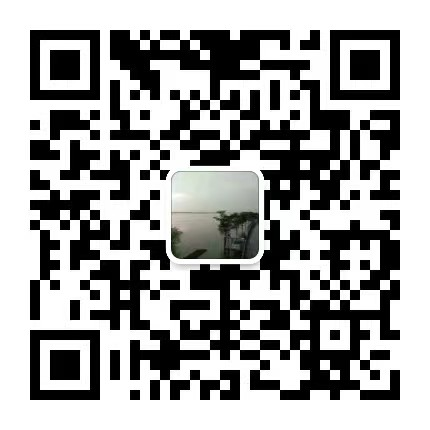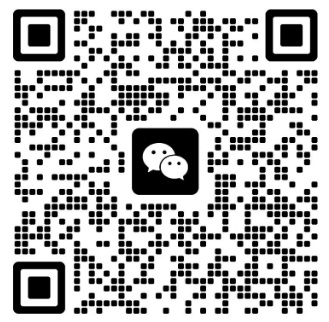## 甘肃成人高考高起点《数学》模拟卷一

2021-12-21 09:19:21   来源：admin    点击： 0 微信咨询+ 加入微信交流群+ 加入1.设集合A={a,b,c,d,e}  B={a,b,e},则AUB=(            )
A {a,b,e }  B {c,d} C {a,b,c,d,e}  D2.下列函数为偶函数的是（　　　）
Ay=-x  B y=xsinx  C y=xcosx  D y=x2+x
3.条件甲x=2,条件乙：x2-3x+2=0，则条件甲是条件乙的（　　　）
A　充要条件　　B必要不充分条件　　C充分但不必条件　　D既不充分又不必要条件
4.到两定点A（-1,1）和B（3,5）距离相等的点的轨迹方程为（　　　）
A x+y-4=0  B x+y-5=0  C x+y+5=0  D x-y+2=0
5.两条平行直线z1=3x+4y-5=0与Z2=6x+8y+5=0之间的距离是（　　　）
A 2   B 3   C
2(1)  D 2(3)
6.以椭圆16(x2)+9(y2)=1上的任意一点（长轴两端除外）和两个焦点为顶点的三角形的周长等于（　）
A 12   B 8+2  C 13   D 18
7.函数y=的定义域是（　　）
A  R   B[0,+∞]    C[-4,-2]   D(-4,-2)
8.抛物线y2=-4x上一点P到焦点的距离为3,则它的横坐标是（　　　）
A -4   B -3   C -2   D -1
9.函数f(x)=sinx+x3(   )
A是偶函数　　B是奇函数　　C既是奇函数，又是偶函数　　D既不是奇函数也不是偶函数
10.=(　　　)
A
4(1)   B 2(1)   C 2(3 )   D 4(3 )
11.掷两枚硬币，两枚的币值面都朝上的概率是（　　　）
A
2(1)   B 4(1)   C 3(1)   D 8(1)
12.通过点（3,1）且与直线x+y=1垂直的直线方程是（　　　）
A x-y+2=0   B 3x-y-8=0   Cx-3y+2=0   Dx-y-2=0
13.已知y=loga(2-ax)在［0,1］上是x的减函数，则a的取值范围是（　　　）
A
9(1)   B (1,2)   C (0,2)   D (2,+ ∞)
14.如果向量a=(3,-2),b=(-1,2)，则(2a+b)·(a-b)等于（　　　）
A 28   B 8   C 16   D32
15.若从一批有8件正品，2件次品组成的产品中接连抽取2件产品（第一次抽出的产品不放回去），则第一次取得次品且第二次取得正品的概率是（　　　）
A
9(1)  B 9(2)   C 45(8)   D 45(16)

16.函数y=(x+1)2+1(x≤1)的反函数是
17.给定三点A(1,0)  B(-1,0)   C(1,2)那么通过点A，并且与直线BC垂直的直线方程是
18.过曲线y=
3(1)x3上一点P(2, 3(8))的切线方程是
19.从球队中随机选出5名队员，其身高分别为（单位：cm）180 188 200　195　187,则身高的样本方差为                 cm2

20.设函数y=f(x)为一次函数，已知f(1)=8,f(2)=-1,求f(11)

21.[an]首项为2,公比为3的等比数列，将此数列的每一项取以3为底的对数构成数列［bn］

22.已知锐角三角形ABC的边长AB=10,BC=8,面积S=32,求AC的长（用小数表示，结果保留小数点后两位）

23.在某块地上种植葡萄，若种50株葡萄藤，每株葡萄藤将产出70kg葡萄，若多种1株葡萄藤，每株产量平均下降1kg，试问在这块地上种多少株葡萄藤才能使产量达到最大值，并求出这个最大值。

24.设A,B两点在椭圆
4(x2)+y2=1上，点M（1, 2(1)）是AB的中点
（1）求直线AB的方程　　（2）若该椭圆上的点C的横坐标为-,求三角形ABC 的面积

### 关注公众号### 考试提醒

2023年甘肃成人高考还有

00